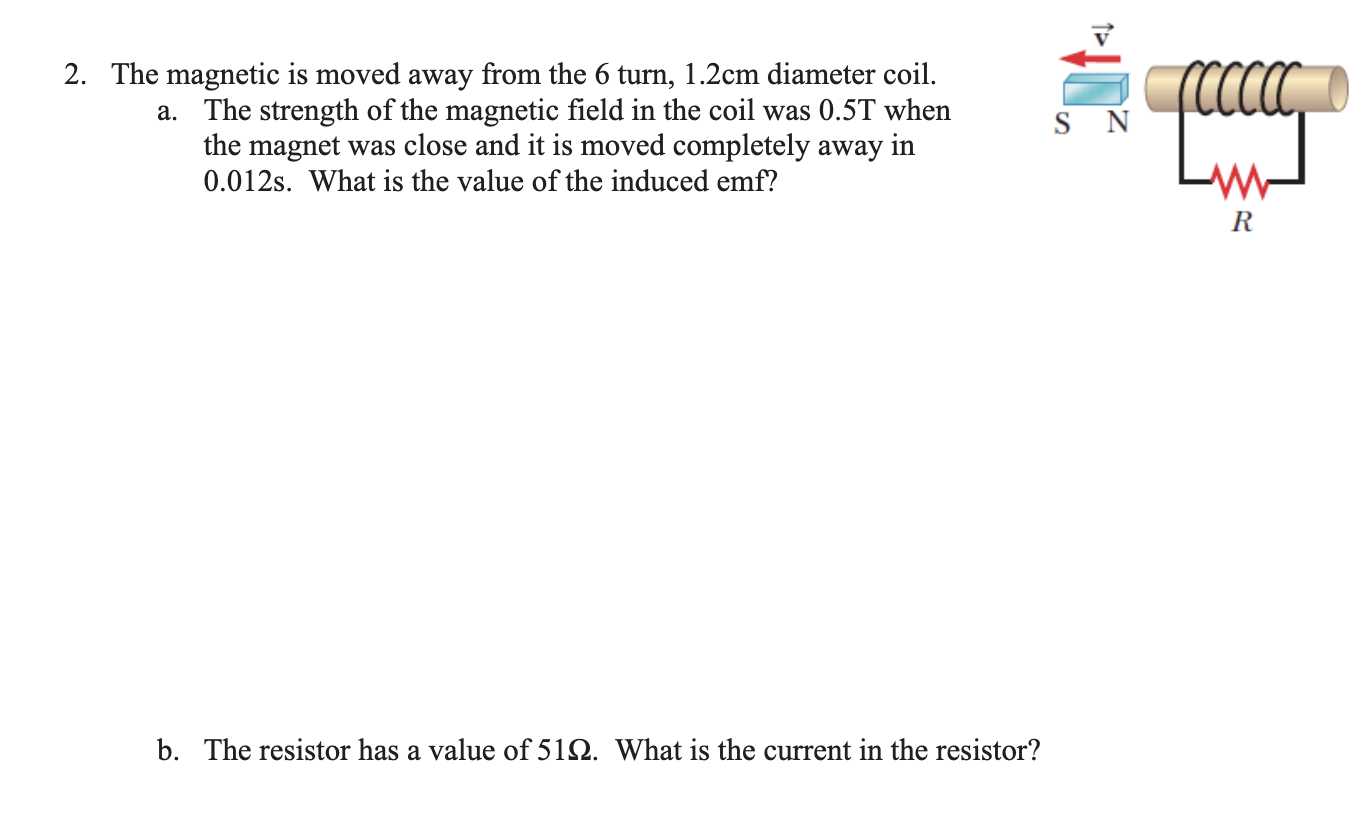Home / Expert Answers / Physics / the-magnetic-is-moved-away-from-the-6-turn-1-2cm-diameter-coil-a-the-strength-of-the-magnetic-fi-pa486

# (Solved): The magnetic is moved away from the 6 turn, 1.2cm diameter coil. a. The strength of the magnetic fi ...

The magnetic is moved away from the 6 turn, 1.2cm diameter coil.

a. The strength of the magnetic field in the coil was 0.5T when the magnet was close and it is moved completely away in 0.012s. What is the value of the induced emf?b. The resistor has a value of 51Ω. What is the current in the resistor? c. Is the direction of the current in the resistor left-to-right or right-to-left?

2. The magnetic is moved away from the 6 turn, diameter coil. a. The strength of the magnetic field in the coil was when the magnet was close and it is moved completely away in . What is the value of the induced emf? b. The resistor has a value of . What is the current in the resistor?

We have an Answer from Expert

Given that,
Number of turns, N = 6 turn
Diameter of coil, d = 1.2 cm =   m
So area of coil is,

Strength of magnetic field when magnet is close to the coil, B1 = 0.5 T
Strength of magnetic field when magnet is moved away from the coil, B2 = 0 T
Time interval, dt = 0.012 s

Here our aim is to find the induced emf in the coil, the current and its direction in the resistor.

We have an Answer from Expert